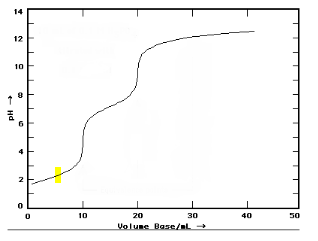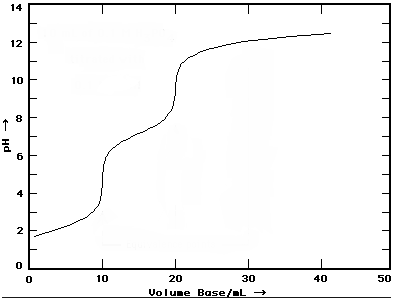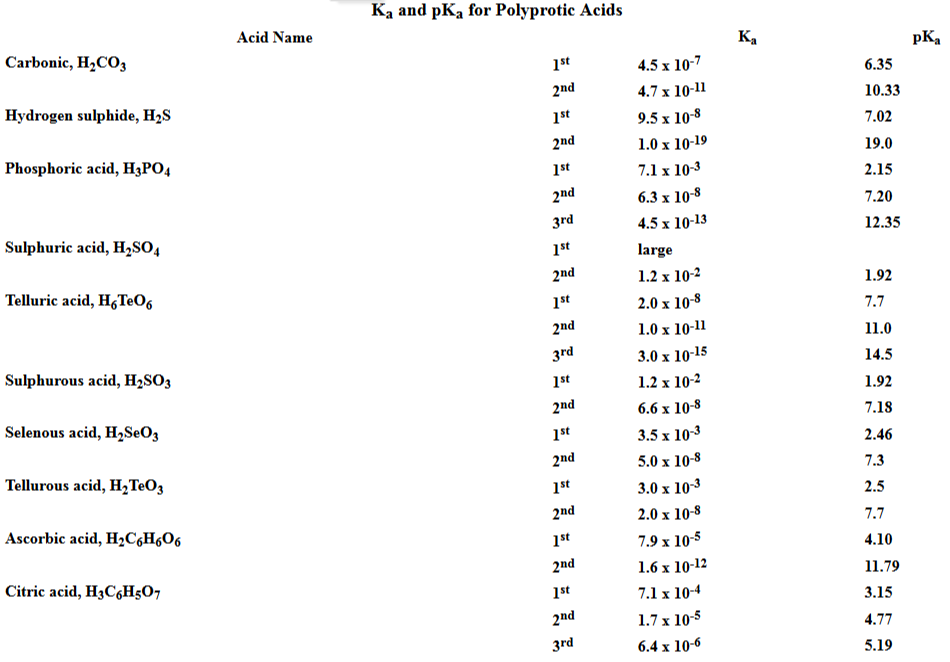# Problem: This is the pH curve for titration of a triprotic acid with NaOH.Only the first two equivalence points can be observed. What is the most likely identity of the acid?Refer to the pKa values provided below.Answer Choices:A. ascorbic acidB. citric acidC. phosphoric acidD. telluric acid

###### FREE Expert SolutionThe pH at volume 1/2 of the volume at equivalence point is equal to pKa hence pKa1 is 2.5.

88% (287 ratings)###### Problem Details

This is the pH curve for titration of a triprotic acid with NaOH.Only the first two equivalence points can be observed. What is the most likely identity of the acid?

Refer to the pKa values provided below.A. ascorbic acid

B. citric acid

C. phosphoric acid

D. telluric acid